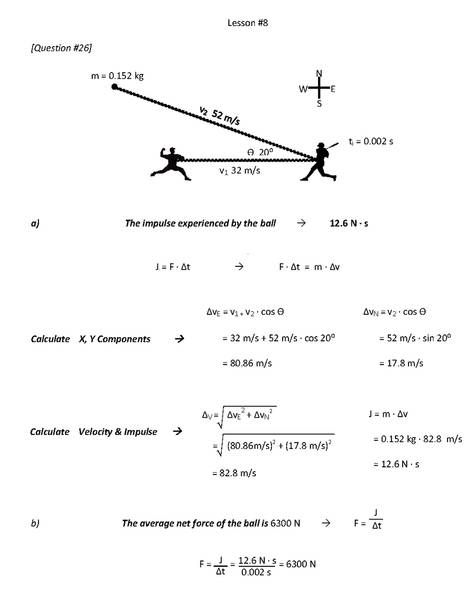# Impulse / 2-D Collisions / Need Clarification?

## Homework Statement

A baseball with a mass of 0.152 kg is moving horizontally at 32.0 m/s [E], when it is struck by a bat for 0.00200 seconds. The velocity of the ball just after the collision is 52.0 m/s [W 20⁰ n].

a) Find the impulse experienced by the ball?

b) Find the average net force of the ball?

J = F ∙ ∆t
F ∙ ∆t = m ∙ ∆v

## The Attempt at a SolutionI've done my work like it says in the text book and it's easy to do when I see examples of similar problems but I still haven't completely wrapped my head around it and I'm not sure why it's right...

Can you please check it over and describe what's going on?

Thank you,
Julian

can you please state a bit more clearly about the point which is troubling you?
why do you think it is not right ?
what is the doubt?

can you please state a bit more clearly about the point which is troubling you?
why do you think it is not right ?
what is the doubt?

I know its right because I read it in the text book, but I wouldn't know what to do if it was a test, and I really wanna ace my exam.

I guess I just don't really understand about the x and y component of velocity and why the hypotenuse of that right triangle is Δv.

What is it the change in velocity because it's not equaling the Δd/Δt of the ball the way I would assume it would? ... Here, this is my problem...

My assumption would be Δv = Δd/Δt 32/1 - 0/0.002- -52/1 = /1 which I know is just wrong, but thats the silly thing that comes to mind when I think about this with no external reference. I know this sounds stupid its just, I guess I'm a noob.

Very Much Appreciated

Also... A triangle with the hypotenuse being the baseballs velocity of 52 m/s leaves an x axis value of 48.9 m/s W and 17.8 m/s E for the y axis. How does the 48.9 relate to Δv ?

haruspex
Homework Helper
Gold Member
Also... A triangle with the hypotenuse being the baseballs velocity of 52 m/s leaves an x axis value of 48.9 m/s W and 17.8 m/s E for the y axis. How does the 48.9 relate to Δv ?
Sounds like you're not drawing the correct triangle.
Set an origin at O. Draw a line E of that to a point A to represent the original velocity vector. Now draw line W 20⁰ N from O to a point B to represent the final velocity vector. The change in velocity is the vector you have to add to OA to get OB; i.e. it is represented by the line from A to B. You want to calculate the length of that line.
How far W of A is B? How far N of A is B?

Sounds like you're not drawing the correct triangle.
The change in velocity is the vector you have to add to OA to get OB; i.e. it is represented by the line from A to B. You want to calculate the length of that line.
How far W of A is B? How far N of A is B?

I realized that the baseball velocity triangle v=52 m/s, vE=-48.9 m/s and ΔvN=17.8 m/s is the v1 of v1 - v2 in Δv --> -48.9 m/s - 32 m/s = -80.9 m/s which is ΔvE. Now I feel comfortable with this problem.

Thanks for everyones input!